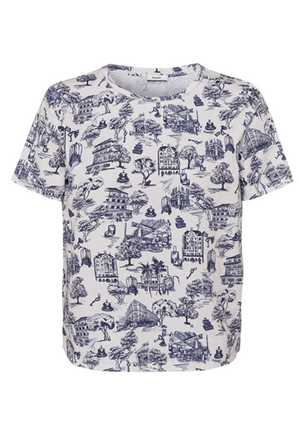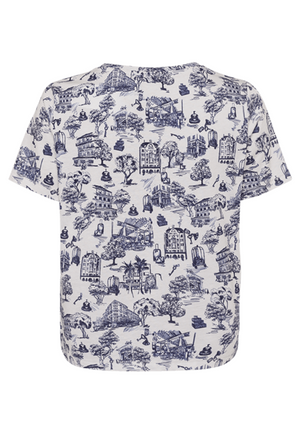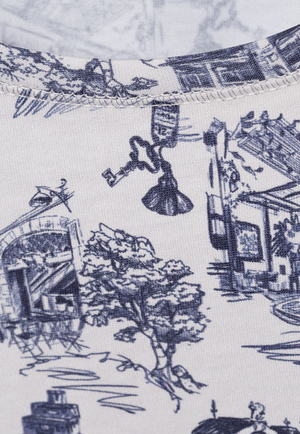Riani

# Print Tee

Regular price \$265 now \$106 Unit price  per

Shipping calculated at checkout.
• 100% cotton
• Machine wash. Hang to dry
• Simple tee
• Fits true to size
• Short sleeve
• Great for casual, work, dressed up or lounging

Size Guide

• 34 = 4 = XS
• 36 = 6 = S
• 38 = 8 = M
• 40 = 10 = M
• 42 = 12 = L
• 44 = 14 =L
• 46 = 16 = XL